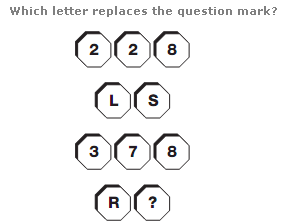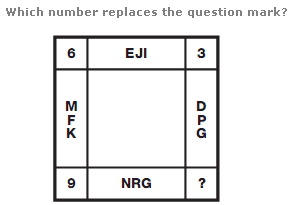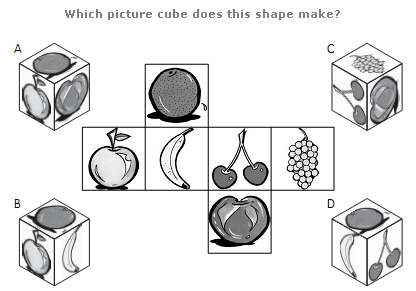# Puzzles - Logical puzzles

Exercise : Logical puzzles
4.:
U
Explanation
:
Multiply the numerical values of the letters in each pair to give the 3 digit result in the spaces above.

5.:
12
Explanation
:
The value at each corner of the diagram equals the difference between the sums of the numerical values of the letters in the boxes adjacent to the corner.

6.